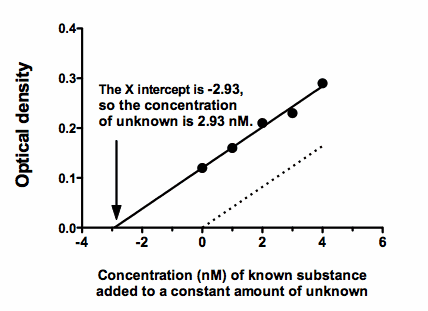## Please enable JavaScript to view this site.

Prism can easily interpolate from a linear or nonlinear standard curve. You perform the assay at a number of known concentrations, fit a line or curve, and interpolate the unknown values.

But there is a problem with interpolating from a standard curve. The results can be incorrect when the unknown sample are contaminated with other substances that alter the assay. This is known as the matrix effect problem.

The Standard Addition Method is a way to bypass this problem. Add various known concentrations (including zero) of known substance to a constant amount of the unknown, and perform the assay. This ensures that all the samples have the same amount of unknown, including any substances that interfere with the assay.

Fit the data with linear regression. The Standard Addition Method only works when the assay measurement (Y) is proportional to the concentration of substance you are measuring.

The dotted line in the figure below shows how the assay results (usually optical density) varies as you change the concentration of the substance you are assaying. The circles and solid line show the assay results in the presence of an unknown concentration of the substance you are assaying. This pushes the line up and to the left.

The value you want to know is how far the solid line is shifted to the left of the dotted line. There is an easy way to find out: Extrapolate the line down to Y=0.  One of the parameters that Prism reports is the X intercept, which will be negative. Take the absolute value, and that is the concentration of the unknown substance. The confidence interval for the X intercept gives you the confidence interval for the concentration of the unknown. Simply multiply both confidence limits by -1.To plot the data in Prism, you'll want to extend the linear regression line to start at an X value equal to the X intercept (a choice in the Linear regression parameters dialog). You may also want to move the origin to the lower left, a choice on the first tab of the Format Axis dialog. Prism file.